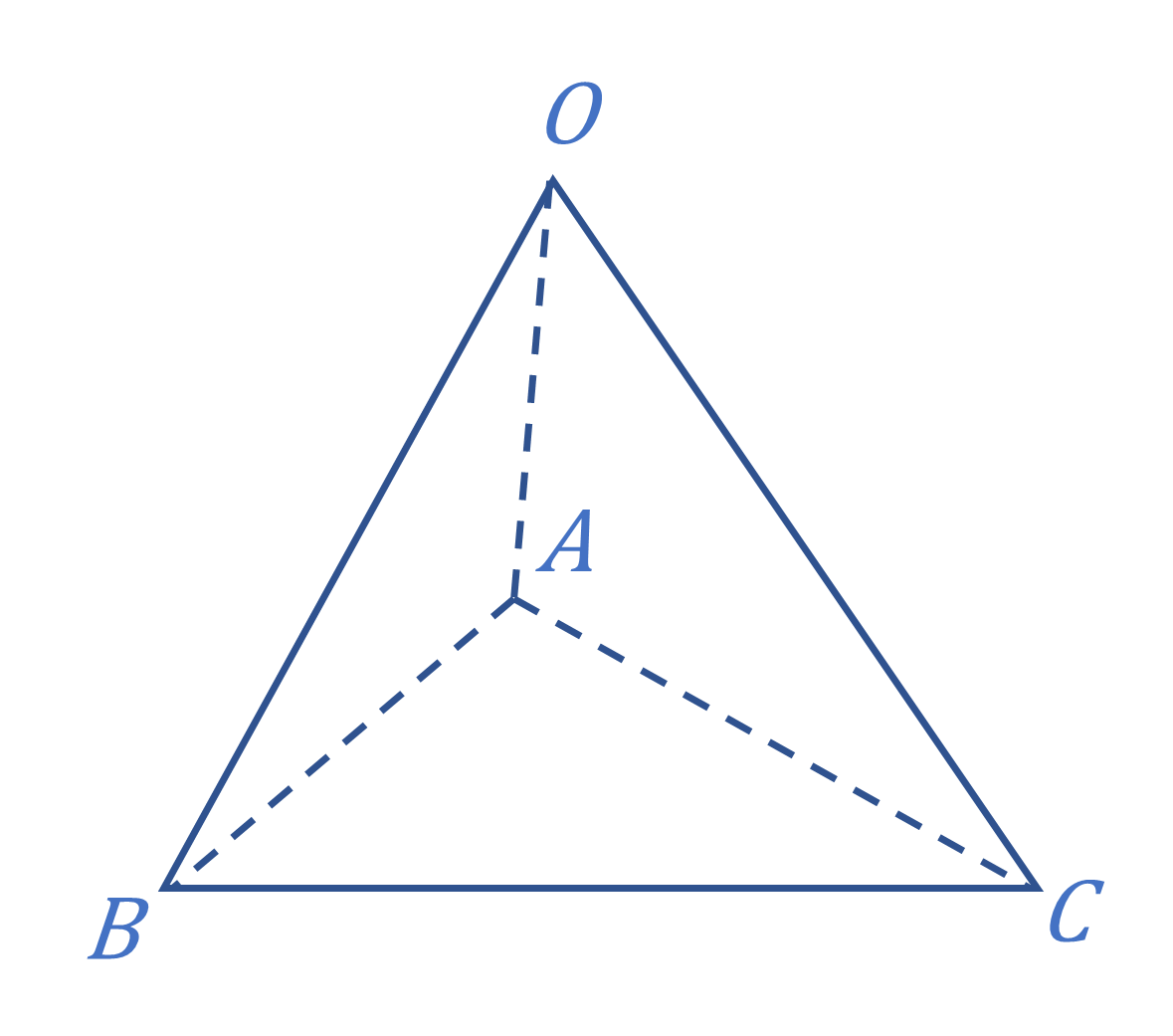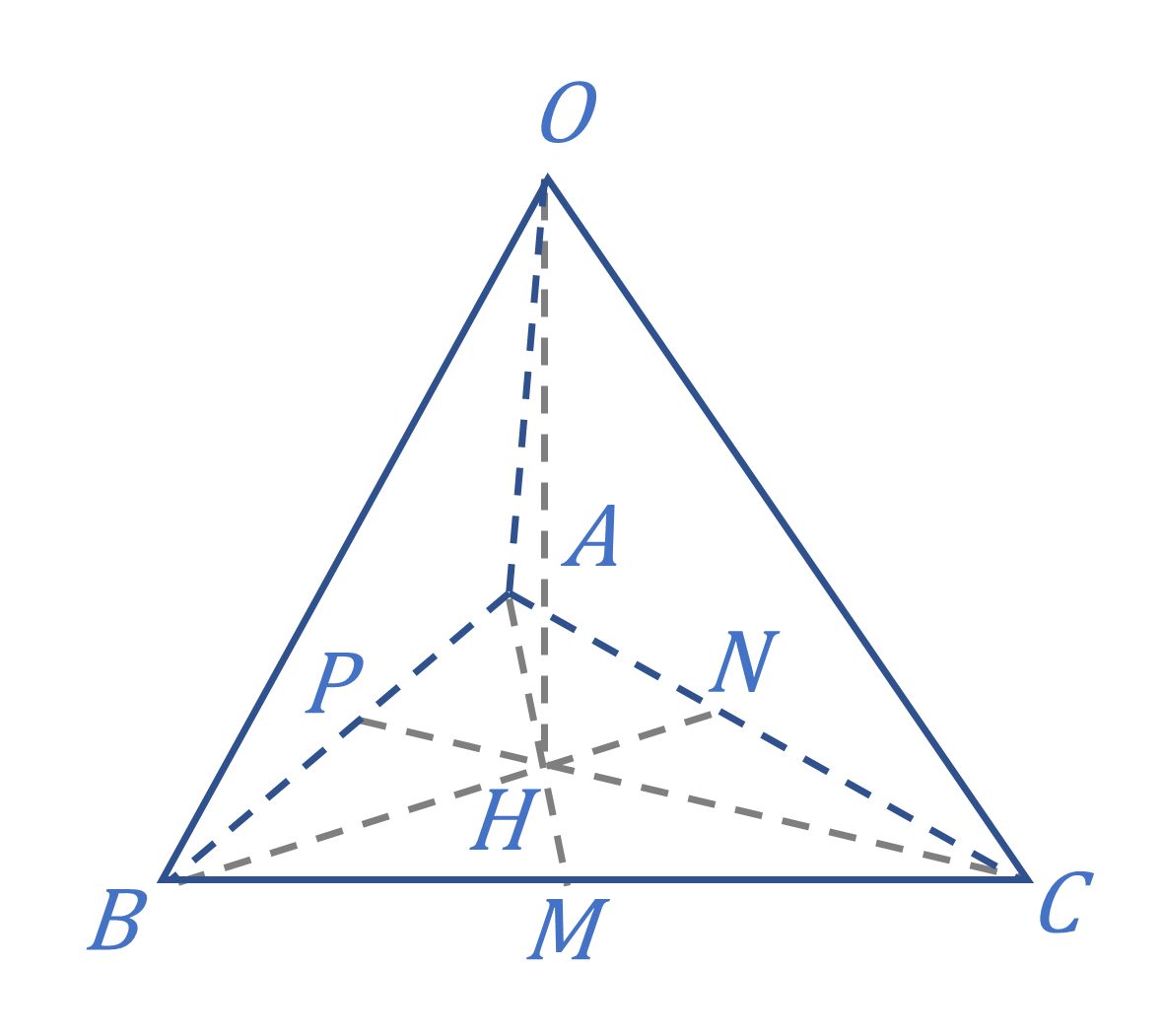# 立体几何和平面几何发生了关系，怎么办？

## 【原题】

1. $O$ から平面 $ABC$ に下るけ垂線の足 $H$ は。

2. $OC \perp AB$## 【翻译与证明】

1. $O$ 作平面 $ABC$ 的垂线，垂足 $H$$\triangle ABC$的垂心。
2. $OC \perp AB$### 证法一：

1. $O$$OH \perp$ 平面 $ABC$，垂足为 $H$
连结 $AH$ 并延长，交 $BC$$M$
显然，$OA$ 在平面 $ABC$ 上的射影是 $AM$
$OA \perp BC$
∴ 由三垂线定理的逆定理可知，$AM \perp BC$，即 $AM$$\triangle ABC$ 的高。
连结 $BH$ 并延长，交 $AC$$N$，类似地，可以证得 $BN$ 也是 $\triangle ABC$ 的高。
$C$$CP \perp AB$
$AM \text{、} BN$ 都是 $\triangle ABC$ 的高，而且交于点 $H$
∴ 由三角形的垂心定理可知，$CP$ 过点 $H$，且 $H$$\triangle ABC$ 的垂心。
2. $OH \perp$ 平面 $ABC$，∴ $OC$ 的射影是 $CP$
又 ∵ $CP \perp AB$ ，由三垂线定理可知，$OC \perp AB$

### 证法二（不使用三垂线定理及其逆定理）：

1. $O$$OH \perp$ 平面 $ABC$，垂足为 $H$
连结 $AH$ 并延长，交 $BC$$M$
$OH \perp$ 平面 $ABC$，且 $BC \subset$ 平面 $ABC$，∴ $OH \perp BC$
又 ∵ $OA \perp BC$，∴ $BC \perp$ 平面 $OAH$
$AM \subset$ 平面 $OAH$，∴ $BC \perp AM$，即 $AM$$\triangle ABC$ 的高。
连结 $BH$ 并延长，交 $AC$$N$，类似地，可以证得 $BN$ 也是 $\triangle ABC$ 的高。
$C$$CP \perp AB$
$AM$$BN$ 都是 $\triangle ABC$ 的高，而且交于点 $H$
∴ 由三角形垂心定理可知，$CP$ 过点 $H$，且 $H$$\triangle ABC$ 的垂心。
2. $OH \perp$ 平面 $ABC$，且 $AB \subset$ 平面 $ABC$，∴ $OH \perp AB$
又 ∵ $CP \perp AB$，∴ $AB \perp$ 平面 $OCH$
又 ∵ $OC \subset$ 平面 $OCH$，∴ $OC \perp AB$

### 证法 3：向量法

$\boldsymbol{a}=\vec{OA}$$\boldsymbol{b}=\vec{OB}$$\boldsymbol{c}=\vec{OC}$

$\vec{OA}\left(\vec{OB}-\vec{OC}\right)+\vec{OC}\left(\vec{OA}-\vec{OB}\right)+\vec{OB}\left(\vec{OC}-\vec{OA}\right)=0$

$\vec{OA}\cdot\vec{CB}+\vec{OC}\cdot\vec{BA}+\vec{OB}\cdot\vec{AC}=0$

$\vec{OC}\cdot\vec{BA} = 0$，即 $OC \perp AB$

$\vec{AH}\cdot\vec{BC}=\left(\vec{OH}-\vec{OA}\right)\cdot\vec{BC}=\vec{OH}\cdot\vec{BC}-\vec{OA}\cdot\vec{BC}=0$

$\vec{BH}\cdot\vec{AC}=\left(\vec{OH}-\vec{OB}\right)\cdot\vec{AC}=\vec{OH}\cdot\vec{AC}-\vec{OB}\cdot\vec{AC}=0$

$\vec{CH}\cdot\vec{AB}=\left(\vec{OH}-\vec{OC}\right)\cdot\vec{AB}=\vec{OH}\cdot\vec{AB}-\vec{OC}\cdot\vec{AB}=0$

$AH \perp BC$$BH \perp AC$$CH \perp AC$

∴ 由三角形垂心定理可知， $H$$\triangle ABC$ 的垂心。

## 图片版权

“我这么可爱，请给我钱 o(*^ω^*)o”https://blog.kukmoon.com/6f7a0357/

Kukmoon谷月

2021年11月6日